# ILP Part 3 – Division, remainder, exponentiation, roots

This is the third part of the ILP series. For your convenience you can find other parts in the table of contents in Part 1 – Boolean algebra

In the first part we defined Boolean algebra operators using ILP. Last time we implemented multiplication. Today we are going to define another basic operators: division and remainder (modulo). We are also going to see how to calculate powers and roots with constant degree.

# Division

We already know how to multiply variables and now we will use this skill to divide numbers. We will perform integer division so the result of the operation will be an integer. Formally: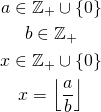We want to divide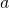by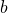and take only integer part of the result. You probably remember from school that division can be seen as an inverted multiplication and we will use this intuition. The result is a maximum possible number that multiplied byis not greater that. Formally: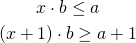You might wonder why we need to addin the second inequality but this is required when we try to calculate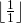.

# Remainder

Previously we ignored the non-integer part of the division result, however, we might want to use this amount which is left over. In fact this is rather easy. First, let’s define the actual number we would like to calculate:We can obtain the result by dividing the numbers and then multiplying them back and subtracting. Since the division ignores the remainder, it will not be included in multiplication and we will be able to extract it using subtraction. See: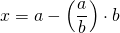Of course here we perform the integer division defined in previous section.

# Power

In this section we will calculate the power of a number, however, we will only consider constant exponent. In one of the next posts we will see how to calculate powers using variable as an exponent, however, for now we will stick to constant value.
Let’s calculate: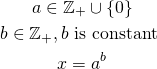This can be seen as a multiplication performedtimes. For instance, to calculate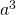we can calculate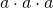and this is the result. No magic this time.

# Roots

Using similar concept as with division, we can calculate root of constant degree. Basically we are looking for a number which raised to the correct power is not greater than the radicand. So: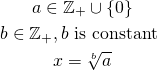The formula is as follows: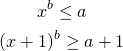Power here is defined as in previous section.

# Summary

Today we have seen how to calculate other basic mathematical operators. For now we are able to use Boolean algebra and perform basic computations. In the next part we will see how to compare numbers — we we will define relational operators. We will get back to powers using variable as an exponent in the next few posts, when we will know how to calculate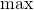and.